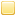##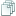Topic: Mathematical Randomness (Read 9221 times)previous topic - next topic

0 Members and 1 Guest are viewing this topic.
Mathematical Randomness
##### July 06, 2013, 06:13:33 pm
Needing a bit more help, on a couple of things.
Hope I'm not a bother, but wanted to ask anyways.
Before testing my Battle script, wanted to test sphere's randomness.

. . .

[Explanation:] Reason for importance:
Choices in-game are sometimes determined through parts of the 1/4th ratio:
1-25... 26-50...  51-75... 76-100.
These ranges are skewed, depending on levels which help determine which random choice is made.
Things like whether attacks are a hit, miss, critical, weak.
The random number should range between 0 - 100.

So wrote this up to test sphere's randomness:

Code: (javascript) [Select]
` var Rnd = 0;Rnd = Math.floor(Math.random() * 100);Abort(Math.floor((Math.random() * 100)  / Rnd));`

[Code_Explanation:] What it's suppose to do is pit one random number against another.
If the random numbers are the same, the answer will come out as "one"
If one of them is doubled, the answer will come out as "two"
And if they're anything else, they'll come out as 0 or something higher than 2

I did some tallying, and figured out:
the most common is 0. Meaning the first number is almost always lower than the second.
-(occurs 7 times for the first 10 counts, and 6 times for the second 10 counts)

Also, the second most common number is 1. Meaning both numbers are the same.
-(occurs 1 time for the first 10 counts, and 2 times for the second 10 counts)

As for 2 or anything higher, both occurred once on each separate counting.

[My question:] Is there anyway to make Math.random() more random?

••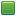• Senior Staff
• Wise Warrior
Re: Mathematical Randomness
##### Reply #1 – July 06, 2013, 07:36:16 pm
No, you can't make Math.random() more random. In fact it's called a 'pseudorandom' number generator. I don't know if there are any cryptographic RNG's out there for JS but I guess you could look for one.
If you use code to help you code you can use less code to code. Also, I have approximate knowledge of many things.

Sphere-sfml here
Sphere Studio editor here

Re: Mathematical Randomness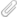##### Reply #2 – July 06, 2013, 08:18:52 pm
Alright, searched the web and found this:

Quote
"Nope JavaScript Math.random() function is not a cryptographic-grade random number generator.
You are better of using the JavaScript Crypto Library's Fortuna implementation which is a strong pseudo-random number generator;
(have a look at the src/js/Clipperz/Crypto/PRNG.js)" -Teoman Soygul

https://www.clipperz.com/open_source/javascript_crypto_library/

Is this what is being referenced?

There's also this: http://www-cs-students.stanford.edu/~tjw/jsbn/

. . .

I'm going through the PRNG.js file right now.
The script is a little bit over my head though.
Attached it if you wanna take a quick peak.

Also, can anyone explain the purpose of "try and throw" in Javascript.
It's being used within the first couple lines, and I have no idea what's going on.

Code: (javascript) [Select]
` try { if (typeof(Clipperz.ByteArray) == 'undefined') { throw ""; }} catch (e) {	throw "Clipperz.Crypto.PRNG depends on Clipperz.ByteArray!";}  try { if (typeof(Clipperz.Crypto.SHA) == 'undefined') { throw ""; }} catch (e) {	throw "Clipperz.Crypto.PRNG depends on Clipperz.Crypto.SHA!";}  try { if (typeof(Clipperz.Crypto.AES) == 'undefined') { throw ""; }} catch (e) {	throw "Clipperz.Crypto.PRNG depends on Clipperz.Crypto.AES!";}  if (typeof(Clipperz.Crypto.PRNG) == 'undefined') { Clipperz.Crypto.PRNG = {}; }`

The Mozilla site talks about "throw" being an exception.
Is that kinda like the "||" operator for "or"?

••• Senior Staff
• Wise Warrior
Re: Mathematical Randomness
##### Reply #3 – July 06, 2013, 08:25:51 pm
Try...catch...throw (and finally) are for error handling.

Code: (javascript) [Select]
`try {  var t = 5 + NaN;}catch(e) {  throw "Not a valid add\n" + e; // e encodes the actual error message}`

It's slower to use try...catch everywhere, but useful for making sure certain portions of the code run without crashing everything (by catching the error you can attempt to course-correct or display a more thorough error message).

The PRNG library was just checking to see if those features are installed or not. If a feature wasn't installed it would throw an error. The error is then caught and displayed to the screen. I'll take a peak at PRNG later and see how it's like.
If you use code to help you code you can use less code to code. Also, I have approximate knowledge of many things.

Sphere-sfml here
Sphere Studio editor here

Re: Mathematical Randomness##### Reply #4 – July 06, 2013, 09:04:11 pm
Alright, so it's more like when Sphere finds a problem and the script and returns with a message...
Or more like the Abort() function in an if-then conditional where the message is created by the user, and aborts if it can't find the installed features?

I think I got it.
And cool man, feel free to take your time.

. . .

Quote

A lot of programs (like video games) will actually use different processes on your machine to determine a random number,
like the number of milliseconds until the next second on your computer's clock or find other sources.

The "random numbers" generated by a computer are actually pseudo-random numbers.
They're entirely predictable if you know what function was used to generate them, and what input was given to the function.
For example, I once wrote a "Linear Congruential Generator", which is a very simple pseudo-random number generator.
All it does is take in a seed number to start with and perform this simple mathematical function on it:

Code: [Select]
` f = modulus((ax + c), m) `

"f" is your new random number, "x" is the seed number, "a" and "m" are constants with supposedly some neat mathematical properties.
To make a new random number, you take the previous result as the new seed and run the function again.
This will output a series of numbers within a specified range (0 to m) that have some of the useful properties of truly random numbers.

Looking up some more stuff on Cryptography and found this.
While looking at the script, I inferred that the library is pulling values (numbers) from multiple sources (clock, mouse, keyboard).
As indicated here (line 800 - 845):

Code: (javascript) [Select]
` _clipperz_crypt_prng_defaultPRNG = null;Clipperz.Crypto.PRNG.defaultRandomGenerator = function() {	if (_clipperz_crypt_prng_defaultPRNG == null) {		_clipperz_crypt_prng_defaultPRNG = new Clipperz.Crypto.PRNG.Fortuna();		//.............................................................		//		//		TimeRandomnessSource		//		//.............................................................		{			var newRandomnessSource;					newRandomnessSource = new Clipperz.Crypto.PRNG.TimeRandomnessSource({intervalTime:111});			_clipperz_crypt_prng_defaultPRNG.addRandomnessSource(newRandomnessSource);		}		//.............................................................		//		//		MouseRandomnessSource		//		//.............................................................		{			var	newRandomnessSource;						newRandomnessSource = new Clipperz.Crypto.PRNG.MouseRandomnessSource();			_clipperz_crypt_prng_defaultPRNG.addRandomnessSource(newRandomnessSource);		}		//.............................................................		//		//		KeyboardRandomnessSource		//		//.............................................................		{			var	newRandomnessSource;						newRandomnessSource = new Clipperz.Crypto.PRNG.KeyboardRandomnessSource();			_clipperz_crypt_prng_defaultPRNG.addRandomnessSource(newRandomnessSource);		}	}	return _clipperz_crypt_prng_defaultPRNG;};`

How it's pulling those values, and what it's doing with them I can't figure out.
I'm guessing, referring to the quoted comment above, that it's using the values pulled as seeds.
And then using those seeds in a mathematical formula to create the random number.
What the formula is, and where the function is to bring it all together, again I've yet to figure out.

[Edit:] Also, there's another script/library it keeps referencing, thought I'd might attach it too. Just in case it's needed.

••• Senior Staff
• Wise Warrior
Re: Mathematical Randomness
##### Reply #5 – July 06, 2013, 09:13:28 pm
Hmm those are a bit too much. I found another better alternative that even implements Dungeons and Dragons dice rolls which is very useful for RPG's.

https://github.com/skeeto/rng-js

It's also easy to implement into existing game code:
Code: (javascript) [Select]
`Math.random = RNG.prototype.uniform.bind(new RNG('my seed'));`

One issue: it might use new JS features that are not in Sphere v1.5 and 1.6. So I'll have to check and see what's needed. For example the above uses .bind() which was adapted late into JS. You can go around that by this code somewhere in your code files to add in .bind():

Code: (javascript) [Select]
`if (Function.prototype.bind === undefined) {    Function.prototype.bind = function (self) {        var args = Array.prototype.slice.call(arguments, 1), func = this;        return function () {            return func.apply(self, args.concat(Array.prototype.slice.call(arguments)));        };    };}`
If you use code to help you code you can use less code to code. Also, I have approximate knowledge of many things.

Sphere-sfml here
Sphere Studio editor here

Re: Mathematical Randomness
##### Reply #6 – July 06, 2013, 09:58:56 pm
[Question:]
Does Math.random now replace the old instance of Math.random(),
or should I replace "Math.random" with another name for a variable?

And to call it, do I still use Math.random() as usual?
Unless named otherwise?

[Edit:] Lol, nvm. It explains right on the Git page.

••• Senior Staff
• Wise Warrior
Re: Mathematical Randomness
##### Reply #7 – July 06, 2013, 10:14:40 pm
Well of course! What else could that line of code do? It literally sets (replaces) Math.random with the new implementation, like setting any other variables in JS.

Well I think the modification I put on my last post is all you need to use RNG-js in your game code.
If you use code to help you code you can use less code to code. Also, I have approximate knowledge of many things.

Sphere-sfml here
Sphere Studio editor here

Re: Mathematical Randomness
##### Reply #8 – July 06, 2013, 10:17:38 pm
Getting an error though, telling me "RNG.prototype.uniform has no properties"
Was the "Function" before prototype meant to be capitalized?

Attempted to lowercase them, then it demanded parentheses and brackets, which I place but still doesn't work.
Kinda drove me clueless.

Simply called it like this:

Code: [Select]
` Abort(Math.random());`

••• Senior Staff
• Wise Warrior
Re: Mathematical Randomness
##### Reply #9 – July 06, 2013, 10:22:55 pm
Hey man it's not that hard to add to your game, this is a quick code example:
Code: (javascript) [Select]
`RequireScript("rng-js.js");if (Function.prototype.bind === undefined) {    Function.prototype.bind = function (self) {        var args = Array.prototype.slice.call(arguments, 1), func = this;        return function () {            return func.apply(self, args.concat(Array.prototype.slice.call(arguments)));        };    };}Math.random = RNG.prototype.uniform.bind(new RNG('my seed'));Abort(Math.random());`

Notice you need to copy the RNG-js code into a separate file and save it as 'rng-js.js' in the /scripts folder for this to work.
If you use code to help you code you can use less code to code. Also, I have approximate knowledge of many things.

Sphere-sfml here
Sphere Studio editor here

••• Senior Staff
• Wise Warrior
Re: Mathematical Randomness
##### Reply #10 – July 06, 2013, 10:24:48 pm
I'll perform no better than your tests with Math.random(), but you will notice that because it is seeded you'll get the same answers each time you run it.
If you use code to help you code you can use less code to code. Also, I have approximate knowledge of many things.

Sphere-sfml here
Sphere Studio editor here

Re: Mathematical Randomness##### Reply #11 – July 06, 2013, 10:35:20 pm
Scratch that :p figured it out. Apologies for the run around bro.
I forgot that I copied to the wrong script at first, and forgot to delete the old copy.
And yes, it works fine. Thanks a million man.

• casiotone
•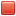Re: Mathematical Randomness
##### Reply #12 – August 14, 2013, 04:33:13 pm
BTW!

You should note that your testing methodology is meaningless and the sample size is also far too small - this is not the correct way to test randomness.

I made a simple test script to generate 10MB of random data:

Code: [Select]
`function game() {	var f = OpenRawFile("prng.out", true);	for (var i = 0; i < 10 * 1024 * 1024; i++) {		f.write(CreateByteArrayFromString(String.fromCharCode(Math.random() * 255)));	}}`

Caveats: Math.random returns a floating point number between 0 and 1, but I want raw bytes, so I convert. This probably isn't perfect.

The 'ent' tool is useful for measuring randomness: http://fourmilab.ch/random/

It takes an input file and will run a number of tests.

Code: [Select]
`\$ ent prng.out Entropy = 7.988667 bits per byte.Optimum compression would reduce the sizeof this 10444516 byte file by 0 percent.Chi square distribution for 10444516 samples is 82493.96, and randomlywould exceed this value less than 0.01 percent of the times.Arithmetic mean value of data bytes is 127.5418 (127.5 = random).Monte Carlo value for Pi is 3.155868268 (error 0.45 percent).Serial correlation coefficient is -0.000395 (totally uncorrelated = 0.0).`

We can see that the results are that: the Chi square test shows it isn't truly random (this is to be expected as it is a PRNG) but it does have high entropy, gives a good distribution and low serial correlation. You can check the website for ent for a better explanation of these values.

For comparison, here is the output on 10MB of data from /dev/urandom
Code: [Select]
`\$ ent urandom.out Entropy = 7.999982 bits per byte.Optimum compression would reduce the sizeof this 10485760 byte file by 0 percent.Chi square distribution for 10485760 samples is 257.28, and randomlywould exceed this value 44.82 percent of the times.Arithmetic mean value of data bytes is 127.4865 (127.5 = random).Monte Carlo value for Pi is 3.141253335 (error 0.01 percent).Serial correlation coefficient is -0.000208 (totally uncorrelated = 0.0).`

And the output for /dev/random:

Code: [Select]
`\$ ent random.out Entropy = 7.999983 bits per byte.Optimum compression would reduce the sizeof this 10485760 byte file by 0 percent.Chi square distribution for 10485760 samples is 245.35, and randomlywould exceed this value 65.65 percent of the times.Arithmetic mean value of data bytes is 127.5263 (127.5 = random).Monte Carlo value for Pi is 3.139946419 (error 0.05 percent).Serial correlation coefficient is -0.000122 (totally uncorrelated = 0.0).`

As expected these two have a better chi square distribution as they use hardware entropy sources.

I believe that the version of SM used in Sphere is using a linear congruential generator ripped from Java.

Anyway, a conclusion:

Unless you're doing cryptography in Sphere, the built in Math.random implementation really is good enough.

• Fat Cerberus
••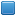• Global Moderator
• Sphere Developer
Re: Mathematical Randomness
##### Reply #13 – August 14, 2013, 09:57:44 pm

Anyway, a conclusion:

Unless you're doing cryptography in Sphere, the built in Math.random implementation really is good enough.

Indeed it is in a lot of cases; however, I don't think that was the crux of the argument here.  The main issue with Math.random() in JS is there's no way to manually seed it, the JS engine automatically seeds it with the current time and there's nothing you can do about it.  If you ever need a custom seed (as is often the case with games), you're stuck using a custom generator.
Sphere 5.6.2 - neoSphere engine - Cell compiler - SSj debugger
•